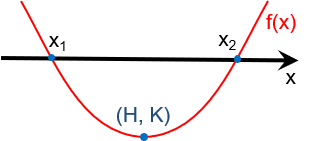Created by Krishna Nelaturu
Last updated: Jul 11, 2022

Welcome to our quadratic formula calculator, where you can easily calculate the roots of a quadratic equation. This quadratic formula solver can even find complex roots if they exist! In this article, we shall discuss how to solve quadratic equations using the quadratic formula, so stick around to learn more!

A quadratic equation is a second-degree polynomial equation, meaning that the largest power of the variable $x$ must be 2. The standard form of this quadratic equation is:

$Ax^2 + Bx + C = 0$

where:

• $x$ - The variable in the equation;
• $A$ - The non-zero coefficient of the term $x^2$;
• $B$ - The coefficient of the term $x$; and
• $C$ - The constant term in the equation.

Quadratic equations describe parabolas. You can check out how they look on a graph using our polynomial graphing calculator.

🙋 We call the solutions to a polynomial equation its roots. A quadratic equation can have two roots.

There are other nonstandard forms, which we must first rearrange and reduce to the standard form. This quadratic function calculator accepts two such forms. If you can reduce your equation to the standard quadratic equation form, you can easily calculate its roots using the quadratic formula:

$x = \frac{-B \pm \sqrt{B^2 - 4AC}}{2A}$

For example, lets calculate the roots of the quadratic equation $2x^2 + 4x - 6 = 0$:

• Extract the coefficients. $A = 2$, $B = 4$, and $C = -6$.
• Find the value of the square-root term $B^2 - 4AC$. $4^2 - 4 \times 2 \times -6 = 64$. Taking square-root, we get $8$.
• The first root is:
$\qquad x_1 = \frac{-4 + 8}{2\times2} = 1$
• The second root is:
$\qquad x_2 = \frac{-4-8}{2\times2} = -3$
• You can verify this with our quadratic equation calculator.

## Real and complex roots

Due to the nature of a square root, the square root term in the quadratic formula is crucial in determining whether the quadratic equation will have real roots or complex roots. Aptly, we call it the discriminant term (NOT to be confused with determinant!).

• If $B^2-4AC >0$, the quadratic equation will have real and distinct roots;

• If $B^2 - 4AC = 0$, the quadratic equation will have a real but redundant root, equal to $\frac{-B}{2A}$; and

• If $B^2 - 4AC <0$, the quadratic equation will have distinct complex roots.

For example, let's calculate the roots of the quadratic equation $2x^2 + 4x + 6 = 0$.

• The discriminant term is $4^2 - 4 \times 2 \times 6 = -32$. Hence the quadratic equation should have complex roots.
• The first complex root is:
\qquad\begin{align*} x_1 &= \frac{-4 + \sqrt{-32} }{4}\\ &= \frac{-4 + \sqrt{-32} }{4}\\ &= \frac{-4 + i\sqrt{16\times2} }{4}\\ &=\frac{-4 + 4i\sqrt{2} }{4}\\ x_1 &= -1 + i\sqrt{2} \end{align*}
• Similarly, the second root would be $x_2 = -1 - i\sqrt{2}$.

## How to use this quadratic formula calculator

This quadratic formula calculator will not only calculate the roots of the quadratic equation but can also show you the steps involved! Here is how you unlock the awesomeness of our quadratic formula solver:

• Choose between the following three forms in which you can enter your quadratic equation in our quadratic function calculator:

• Ax2 + Bx + C = 0.
• A(x-H)2 + K = 0.
• A(x-x1)(x-x2) = 0.
• Enter the proper values of the coefficients and constants involved.

• If the discriminant is positive, this quadratic equation calculator will automatically give you the roots of the quadratic equation along with important steps.

• If the discriminant is negative, this quadratic equation solver will calculate the complex roots automatically only if you select  yes in the allow negative discriminant field. Otherwise, it will display a warning message and wait for your approval.

Krishna Nelaturu
Select formula and enter parameters
Formula form
Ax² + Bx + C = 0
A
B
C
Results
Allow negative discriminant
No
Discriminant (Δ)
Standard form: f(x) = Ax² + Bx + C
Vertex form: f(x) = A(x - H)² + K
Factored form: f(x) = A(x - x₁)(x - x₂)People also viewed…

### Pythagorean theorem

Use this Pythagorean theorem calculator to find any of the sides of a right triangle